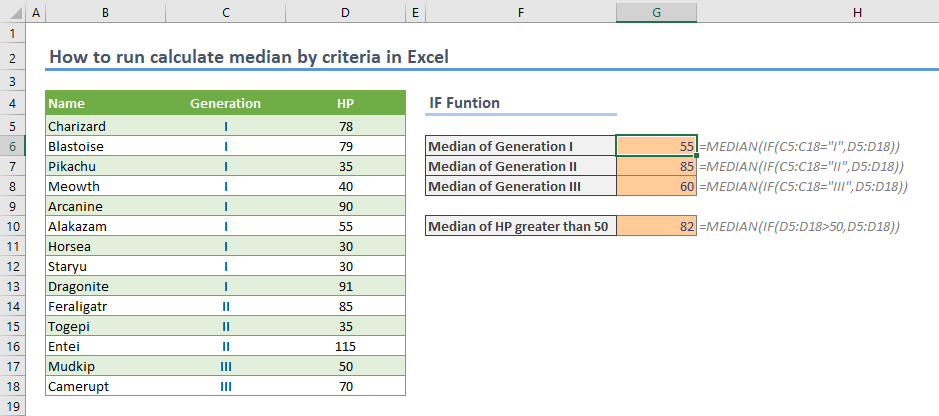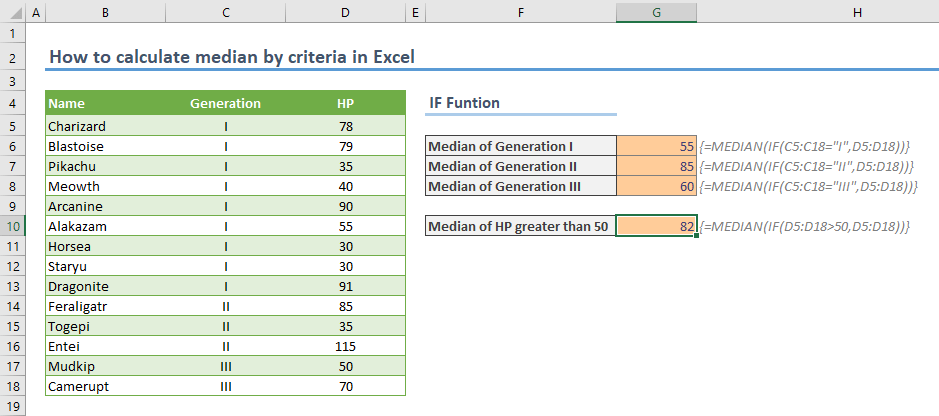In this guide, we're going to show you how to run calculate median by criteria in Excel.

To calculate median based on a criteria, you need to supply a range of values to be compared against the specific criteria defined in the MEDIAN function. Essentially, you can use either of these formulas:

Both functions can return an array of values with a similar syntax. If you don't have access to the FILTER function, you can use the IF function for creating the condition by using it within an array formula. Let’s see both methods on an example:

## To calculate median by criteria with the FILTER function

=MEDIAN(FILTER(<data>,<criteria>))

The FILTER function can filter a range by a specified condition. You need to supply the data range and a logical condition to defined the criteria. For example, the following example formula calculates the median of values under HP that belong to Generation I.The C5:C18="I" logical test is the criterion.

=MEDIAN(FILTER(D5:D18,C5:C18="I"))

The next example is to calculate the median of values greater than 50. You need to use the data range for the criteria argument.=MEDIAN(FILTER(D5:D18,D5:D18>50))

You can check out the FILTER functions page to see more examples, such as using it with multiple criteria.

## IF Function

=MEDIAN(FILTER(<criteria>,<data>))

The only difference aside from formula name is the order of the arguments.Median of Generation I:                 =MEDIAN(IF(C5:C18="I",D5:D18))

Median of HP greater than 50:    =MEDIAN(IF(D5:D18>50,D5:D18))

## Not Microsoft 365 Users

Even if you are not a Microsoft 365 user, you can still calculate median by criteria using the IF function. However, you must submit these formulas as an array formula. To do this, press the Ctrl + Shift + Enter keys after typing in your formula -  do not use the Enter key alone as you normally would. If you press the key combination correctly (at the same time), you will see Excel put curly brackets around your function, and give you the correct result.